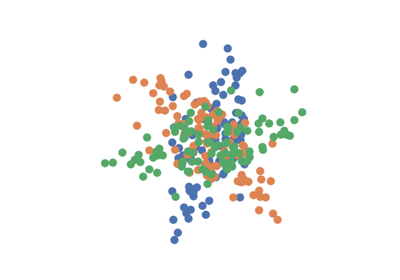# Hotelling¶

class hyppo.ksample.Hotelling

Hotelling $$T^2$$ test statistic and p-value.

Hotelling $$T^2$$ is 2-sample multivariate analysis of variance (MANOVA) and generalization of Student's t-test in arbitary dimension 1.

Notes

The test statistic is formulated as below 2:

Consider input samples $$u_i \stackrel{iid}{\sim} F_U$$ for $$i \in \{ 1, \ldots, n \}$$ and $$v_i \stackrel{iid}{\sim} F_V$$ for $$i \in \{ 1, \ldots, m \}$$. Let $$\bar{u}$$ refer to the columnwise means of $$u$$; that is, $$\bar{u} = (1/n) \sum_{i=1}^{n} u_i$$ and let $$\bar{v}$$ be the same for $$v$$. Calculate sample covariance matrices $$\hat{\Sigma}_{uv} = u^T v$$ and sample variance matrices $$\hat{\Sigma}_{uu} = u^T u$$ and $$\hat{\Sigma}_{vv} = v^T v$$. Denote pooled covariance matrix $$\hat{\Sigma}$$ as

$\hat{\Sigma} = \frac{(n - 1) \hat{\Sigma}_{uu} + (m - 1) \hat{\Sigma}_{vv} } {n + m - 2}$

Then,

$\text{Hotelling}_{n, m} (u, v) = \frac{n m}{n + m} (\bar{u} - \bar{v})^T \hat{\Sigma}^{-1} (\bar{u} - \bar{v})$

Since it is a multivariate generalization of Student's t-tests, it suffers from some of the same assumptions as Student's t-tests. That is, the validity of MANOVA depends on the assumption that random variables are normally distributed within each group and each with the same covariance matrix. Distributions of input data are generally not known and cannot always be reasonably modeled as Gaussian 3 4 and having the same covariance across groups is also generally not true of real data.

References

1

Harold Hotelling. The Generalization of Student's Ratio. The Annals of Mathematical Statistics, 2(3):360–378, August 1931. doi:10.1214/aoms/1177732979.

2

Sambit Panda, Cencheng Shen, Ronan Perry, Jelle Zorn, Antoine Lutz, Carey E. Priebe, and Joshua T. Vogelstein. Nonpar MANOVA via Independence Testing. arXiv:1910.08883 [cs, stat], April 2021. arXiv:1910.08883.

3

Theodore Micceri. The unicorn, the normal curve, and other improbable creatures. Psychological Bulletin, 105(1):156–166, 1989. doi:10.1037/0033-2909.105.1.156.

4

Stephen M. Stigler. Do Robust Estimators Work with Real Data? The Annals of Statistics, 5(6):1055–1098, November 1977. doi:10.1214/aos/1176343997.

Methods Summary

 Calulates the Hotelling $$T^2$$ test statistic. Hotelling.test(x, y) Calculates the Hotelling $$T^2$$ test statistic and p-value.

Hotelling.statistic(x, y)

Calulates the Hotelling $$T^2$$ test statistic.

Parameters

x,y (ndarray) -- Input data matrices. x and y must have the same number of dimensions. That is, the shapes must be (n, p) and (m, p) where n is the number of samples and p and q are the number of dimensions.

Returns

stat (float) -- The computed Hotelling $$T^2$$ statistic.

Hotelling.test(x, y)

Calculates the Hotelling $$T^2$$ test statistic and p-value.

Parameters

x,y (ndarray) -- Input data matrices. x and y must have the same number of dimensions. That is, the shapes must be (n, p) and (m, p) where n is the number of samples and p and q are the number of dimensions.

Returns

Examples

>>> import numpy as np
>>> from hyppo.ksample import Hotelling
>>> x = np.arange(7)
>>> y = x
>>> stat, pvalue = Hotelling().test(x, y)
>>> '%.3f, %.1f' % (stat, pvalue)
'0.000, 1.0'


## Examples using hyppo.ksample.Hotelling¶